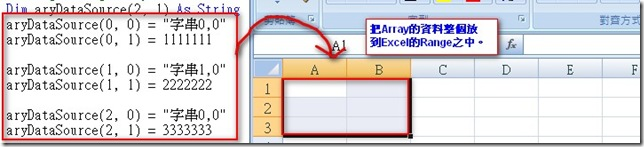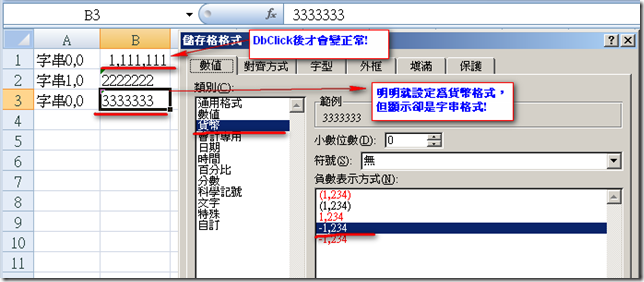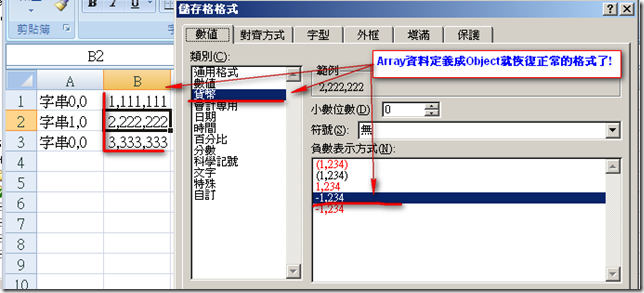### [Excel] Assign Array資料給Range時，要注意Array的型態

[Excel] Assign Array資料給Range時，要注意Array的型態

### 前言

1.一個Cell一個Cell Assign
2.建立檔案，然後用Insert Into的方式(直接從SQL取出資料)
3.設一個Range然後Assign一個Array給它(資料需要加工過，放到Array之中)

...### 研究### 實作

#### 1.建立測試的資料Array

``````Dim aryDataSource(2, 1) As Object
aryDataSource(0, 0) = "字串0,0"
aryDataSource(0, 1) = 1111111

aryDataSource(1, 0) = "字串1,0"
aryDataSource(1, 1) = 2222222

aryDataSource(2, 0) = "字串0,0"
aryDataSource(2, 1) = 3333333``````

#### 2.設定各欄位的資料型態及將資料Array Assign到Range

``````dim strFileNam as string = "c:\test1.xls"
Dim oBook As Object = oExcel.Workbooks.Add
Try
Dim oSheet As Object
oSheet = oBook.Worksheets(1)
'設定Excel各欄位的型態，目前設定字串及數值
'第1欄設成文字
oSheet.columns(1).NumberFormatLocal = "@"
'第2欄設成3位一撇
oSheet.columns(2).NumberFormatLocal = "#,##0"
'傳入Column數，傳出Excel的ColumnLabel
'1=>A, 26=>Z, 27=>AA
Dim strColumnLabel As String = GetExcelColumnLabel(UBound(aryDataSource, 2) + 1)
'將資料Assign給Range
oSheet.Range("A1", strColumnLabel & UBound(aryDataSource, 1) + 1) = aryDataSource
'將資料存檔
oBook.SaveAs(strFileName)
oBook.Close()
oExcel.Quit()

GC.Collect() '強制記憶體回收。
MsgBox("OK!")
Catch ex As Exception
oBook.Close()
oExcel.Quit()
Throw ex
Finally
oBook = Nothing
oExcel = Nothing
End Try``````Hi,